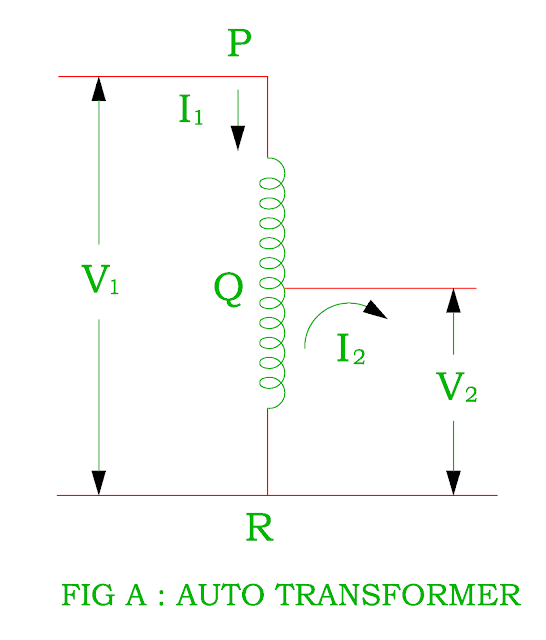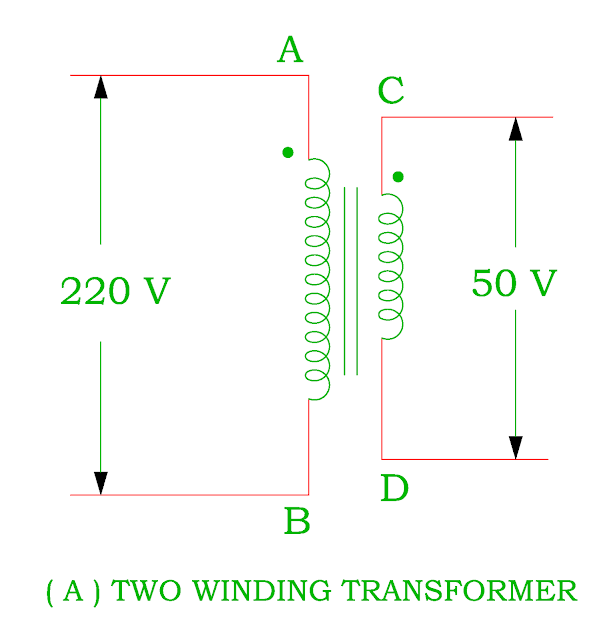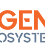## 03/11/2017

### Working of Auto transformer, Saving in Copper Material

• It consists of only single winding which is common to both primary and secondary winding
• It has two end terminals ( PR) acts as primary and one end terminal ( either P or R ) and inter mediate terminal Q acts as a secondary winding ( Figure A ).
• The position of the intermediate terminal Q determines whether auto – transformer works as step down transformer or step up transformer.
• The theory and operation of the auto - transformer is similar to that of conventional two winding transformer
• The auto transformer is cheaper than the ordinary transformer because of single winding uses less copper than the two winding.
• Since the voltage / turn is equal in both winding, the voltage develops across each winding depends on number of turns.• Figure B shows a step down transformer in which PQ winding acts as a primary having N1 turns and QR winding acts as a secondary winding consists of N2 turns.
• If the supply voltage V1 is given to primary winding

Induced emf per turn ( Et ) = V1 / N1
As we know that the value of Et is same for both winding

Secondary voltage V2 = Et × N2

Saving of Copper in Auto transformer as step down transformer
• The weight of winding material ( Copper ) is proportional to the length and area of cross section of the conductors.

W a Volume of material
W a ( a × L )
• The cross section of the conductor depends on current ( I ) and length of conductors is proportional to the number of turns ( N ).
• Therefore the weight of copper is directly proportional to ampere - turns ( NI ).

Weight of the copper in the ordinary transformer ( WO ) = N1I1 + N2I2
Weight of copper in the auto transformer ( Wa ) =
Weight of copper in part PQ a I1 ( N1 – N2 )
Weight of copper in part QR a ( I2 – I1 ) N2
Total weight of copper in the auto transformer a I1 ( N1 – N2 ) + ( I2 – I1 )
N2
a ( I1N1 + I2N2 – 2N2I1 )
Weight of copper in auto transformer ( Wa ) / Weight of copper in ordinary transformer ( WO ) =  ( I1N1 + I2N2 – 2N2I1 ) / ( I1N1 + I2N2 )
= ( I1N1 + I1N1 – 2N2I1 ) / 2N1I1  ( As N1I1 = N2I2 )
= ( 2I1N1 / 2I1N1 ) – ( 2N2I1 / 2I1N1 )
= ( 1 ) – ( K )     ( As K = N2 / N1 = I1 / I2 )
= 1 – K
Therefore Wa = ( 1 – K ) WO
Saving in Copper = WO – Wa
= WO – ( 1 – K ) WO
= KWO

• Therefore the saving in copper material depends on the value of K ( Voltage transformation ratio ).
• Higher value of K, more saving in copper material

Saving of Copper in Auto transformer as Step up transformer

Weight of the copper in the ordinary transformer ( WO ) = N1I1 + N2I2
Weight of copper in the auto transformer ( Wa ) =
Weight of copper in part PR a I2 ( N2 – N1 )
Weight of copper in part QR a ( I– I) N1
Total weight of copper in the auto transformer a I2 ( N2 – N1 ) + ( I– I)
N1
a ( I1N1 + I2N2 – 2N1I2 )
Weight of copper in auto transformer ( Wa ) / Weight of copper in ordinary transformer ( WO ) = ( I1N1 + I2N2 – 2N1I2 ) / ( I1N1 + I2N2 )
= ( I1N1 + I1N1 – 2N1I2 ) / 2N1I1  ( As N1I1 = N2I2 )
= ( 2I1N1 / 2I1N1 ) – ( 2N1I2 / 2 N1I1 )
= ( 1 ) – ( 1 / K )     ( As K = N2 / N1 = I1 / I2 )
= ( 1 – 1 / K )
Therefore W= [ ( 1 – 1 / K ) ] WO
Saving in copper = WO – [ ( 1 – 1 / K ) ] WO
= [ 1 – 1 + 1 / K ] WO
= ( 1 / K ) WO
• Therefore the saving in copper material depends on the value of 1 / K.
• Lower value of K, more saving in copper material
Application
• The auto – transformer is used for starting of synchronous motor and induction motor. Its winding is used only during starting period of the motor for a few seconds.
• It is used as a furnace transformer for getting suitable supply voltage for furnace winding from 230 V supply.
• It is used as laboratory equipment to get suitable supply voltage as per requirement.
• To compensate the small voltage drop in a distribution cable by giving small voltage boost.
• It may be used as interconnecting transformer in 132 kV / 66 kV supply system.
Connection of two winding transformer into Auto – transformer
• The winding connection diagram of an auto transformer from two winding transformer may be as ( I ) Step down transformer ( II ) Step up transformerConversion of Two winding Transformer into Step down Auto Transformer

1.Closed focus transformers could genuinely show up positively in relationship with shell outline transformer for high voltage control transformer applications.

Transformer winding is one more standard bit of a transformer upgrade, since this is the ensured current bearing conductors contorted inside ring.Winding Machine

2.Really I enjoy your site with effective and useful information. It is included very nice post with a lot of our resources.thanks for share. i enjoy this post. text auto reply

3.4.5.This is same as transformer vector diagram

6.This is such a great resource that you are providing and you give it away for free. I love seeing blog that understand the value of providing a quality resource for free. purple amethyst ring

7.I havent any word to appreciate this post.....Really i am impressed from this post....the person who create this post it was a great human..thanks for shared this with us. garnet engagement ring

8.This is a well crafted article, if perhaps just about all bloggers provided the same worth of content while you, the web will be a far better place. Please keep it up! Amethyst Rings

9.Really interesting blog. I’m thankful for this valuable information. Ogen Infosystem is a leading Website Designing and Development Company in Delhi. Also, get SEO and all types of Digital Marketing Services at an affordable price.
Web Development Company

10.A very delightful article that you have shared here about Electrical Enclosures. Your blog is a valuable and engaging article for us, and also I will share it with my companions who need this info. Thankful to you for sharing an article like this.